# Java Program to check Equal Number in Java

In this tutorial you will learn about the Java Program to check Equal Number in Java and its application with practical example.

In this tutorial, we will learn to create a Java Program to check Equal Numbers in Java using java programming.

## Prerequisites

Before starting with this tutorial, we assume that you are best aware of the following Java programming concepts:

• Java Operators.
• Basic Input and Output function in Java.
• Class and Object in Java.
• Basic Java programming.
• If-else statements in Java.

# How do you equal a number in Java?

How to compare integer values are equal or not in Java, we can use either == (operator) or we can simply subtract these two values and values becomes zero means given number are equal. Both are used to compare two values. We can also use the equal() method to find numbers are equal or not.
Example How to check whether they are equal or not, use equal to (== )operator.
a == b

Example2 How to check whether they are equal or not, use the equal to ( )operator.
if(a -b==0)they are equal other wise they are not equal..

## Java Program to check Equal Number in Java

In this program, we will take two values from the user and print numbers are an equal or not the smallest element in an array using a nested for loop. We would first declare and initialized the required variables. Next, we will find the smallest number in an array. Let’s have a look at the code.

Using relational operator equal to (==) in java program..

## Output

Equal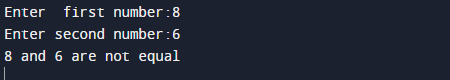Not Equal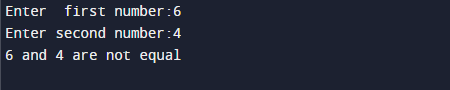using arithmetic operator equal to(-) in java program..

## Output

EqualNot Equal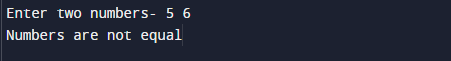In the above program, we have first declared and initialized a set variables required in the program.

• a= it will hold value of first variable.
• b= it will hold value of second variable.
• i and j for iteration
• temp = it will hold temporary value of an array.

After declaring variables we initiate values and take value from user at run time.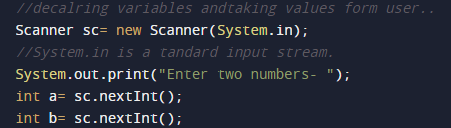then with the help of relational and arithmetic operator we will find given integer are equal or not.

using arithmetic operator equal to(-) in java program..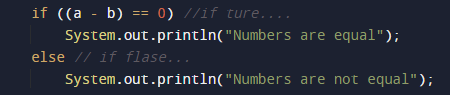Using relational operator equal to (==) in java program..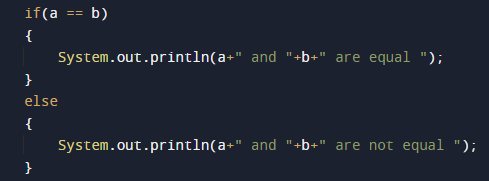and finally we will print the results…

In this tutorial we have learn about the Java Program to check Equal Number in Java and its application with practical example. I hope you will like this tutorial.